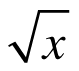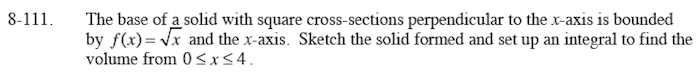### Home > CALC > Chapter 8 > Lesson 8.3.2 > Problem8-111

8-111.

The base of a solid with square cross-sections perpendicular to the x-axis is bounded by f(x) =and the x-axis. Sketch the solid formed and set up an integral to find the volume from 0 ≤ x ≤ 4. Homework Help ✎The base and height of each cross section will be the length of f(x) starting at x = 0 (an impossibly small square) and ending at x = 4 (a 2 x 2 square).